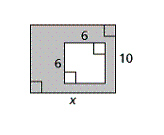Name:    Quiz 1.4-1.5

Multiple Choice
Identify the choice that best completes the statement or answers the question.

Use the Distributive Property and mental math to find the product.

1.

6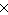82
 a. 498 c. 492 b. 480 d. 486

2.

8(12)
 a. 80 c. 96 b. 88 d. 104

3.

4(17)
 a. 68 c. 64 b. 72 d. 40

Use the Distributive Property to simplify the expression.

4.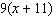a.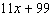c.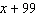b.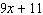d.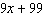5.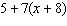a.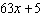c.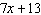b.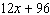d.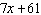Find the value of x that makes the expressions equivalent.

6.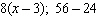a. 32 c. 3 b. 8 d. 7

7.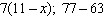a. 11 c. 9 b. 14 d. 7

8.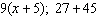a. 72 c. 3 b. 5 d. 9

Use the Distributive Property to rewrite the expression as a product.

9.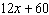a.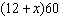c.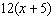b.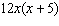d.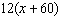10.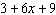a.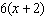c.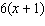b.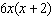d.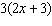Use a formula to find the area of the figure.

11.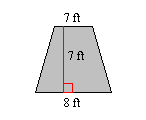a. 105 ft2 c. 52.5 ft2 b. 56 ft2 d. 28 ft2

12.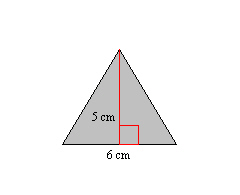a. 11 cm2 c. 30 cm2 b. 15 cm2 d. 36 cm2

13.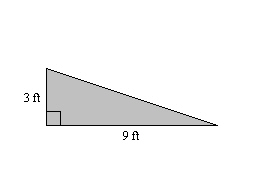a. 24 ft2 c. 13.5 ft2 b. 9 ft2 d. 27 ft2

Numeric Response

Use the Distributive Property and mental math to find the product.

1.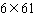Find the value of x that makes the expressions equivalent.

2.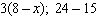3.

A pitcher’s earned run average is the average number of earned runs given up per nine innings. What is the earned run average of a pitcher who gave up 88 earned runs in 264 innings.

Earned Run Average =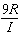, where R is the number of earned runs and I is the number of innings pitched.